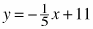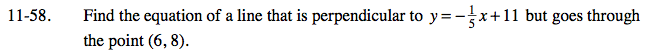### Home > AC > Chapter 11 > Lesson 11.1.5 > Problem11-58

11-58.

Find the equation of a line that is perpendicular tobut goes through the point (6, 8). Homework Help ✎The slope of a perpendicular line is the opposite reciprocal of the slope of the original line.

Use y = mx + b.

y = 5x − 22